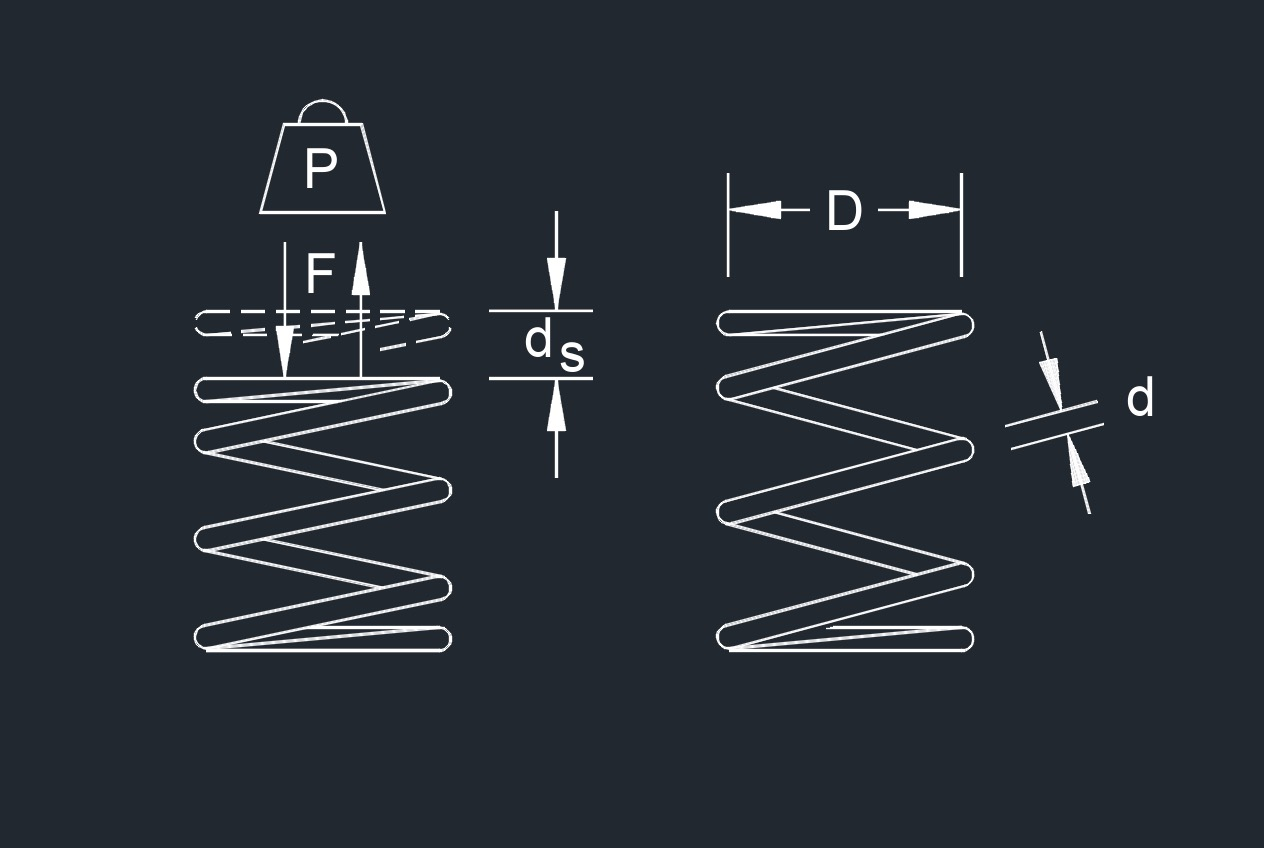# Spring Rate

Written by Jerry Ratzlaff on . Posted in FastenerSpring rate, abbreviated as $$n_s$$, is not the same as spring load.  The spring rate is based on the spring's working load.  Spring rate is the rate of force or weight required to travel one unit of measurement.  The lower the spring rate, the softer the spring.  The softer the spring, the smother the ride.  Spring load is the amount of weight a spring is designed to carry when compressed to a certain height.

## formulas that use Spring Rate

 $$\large{ n_s = \frac{ P }{ d_s } }$$ $$\large{ n_s = \frac{ P \;-\; T_i }{ d_s } }$$ $$\large{ n_s = \frac{ G \; d^4 }{ 10.8 \; D \; n_a } }$$ (rate per 360 degrees) $$\large{ n_s = \frac{ G \; d^4 }{ 8 \; D^3 \; n_a } }$$ (pitch angle is less than 15 degrees or displacement per turn is less than D/4.)

### Where:

$$\large{ n_s }$$ = spring rate

$$\large{ P }$$ = load

$$\large{ D }$$ = mean coil diameter

$$\large{ n_a }$$ = number of active coils

$$\large{ G }$$ = shear modulus of material

$$\large{ d_s }$$ = spring displacement

$$\large{ T_i }$$ = initial tension

$$\large{ d }$$ = wire diameter

Tags: Equations for Spring